Precalculus : Use Odd-Even Identities

Example Questions

Example Question #1 : Fundamental Trigonometric Identities

Which of the following statements is false?Explanation:

Of the six trigonometric functions, four are odd, meaning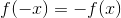. These four are:

• sin x
• tan x
• cot x
• csc x

That leaves two functions which are even, which means that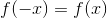. These are:

• cos x
• sec x

Of the aforementioned, only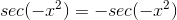is incorrect, since secant is an even function, which implies that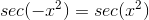Example Question #1 : Use Odd Even Identities

Find the value of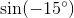.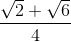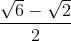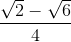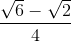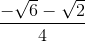Explanation:

Rewrite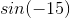by odd and even identities.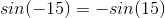Use the difference identity of sine, and choose the special angles 45 and 30, since their difference equals to 15.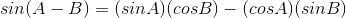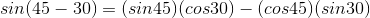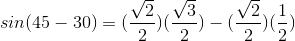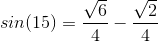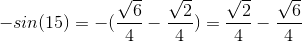Example Question #1 : Use Odd Even Identities

Simplify: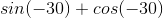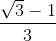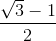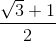Explanation:

Write the even and odd identities for sine and cosine.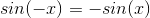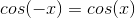Rewrite the expressionand evaluate.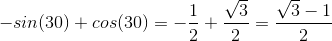Example Question #4 : Fundamental Trigonometric Identities

Simplify: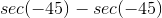Explanation:

In order to simplify, rewrite the expression after applying the rule of odd-even identities for the secant function.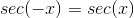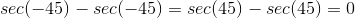All Precalculus Resources